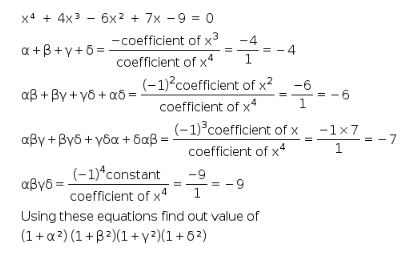# If α,β,γ, and δ are the roots of the equation x⁴ + 4x³ - 6x² + 7x -9 = 0

If α,β,γ, and δ are the roots of the equation x⁴ + 4x³ - 6x² + 7x -9 = 0, then the value of (1+α²) (1+β²)(1+γ²)(1+δ²) is _____.